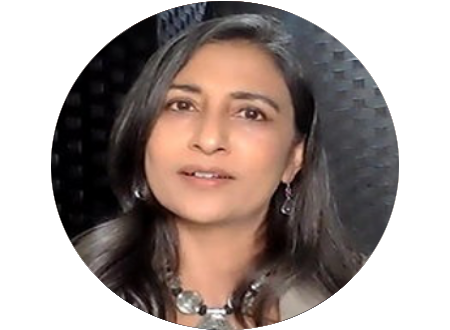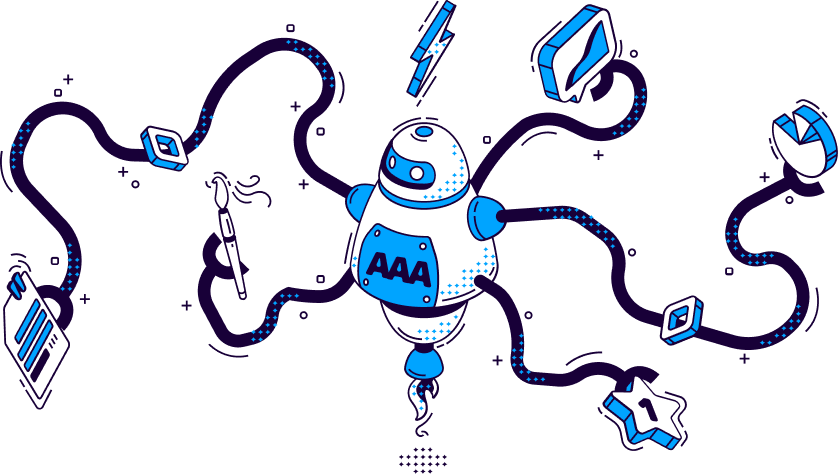# Healthy Minds, Healthy Workplaces

Challenges, adversities, and stressors at work are inevitable. According to statistics, employees have been more frustrated, burnout, and exhausted at work because of overwhelming and emotional stressors.

Do not journey alone as a healthy work-life balance is essential for peak performance. Here at Aventis Wellness, we prioritize your own and your company's mental well-being.

```!function(t,n){"object"==typeof exports&&"undefined"!=typeof module?module.exports=n():"function"==typeof define&&define.amd?define(n):(t="undefined"!=typeof globalThis?globalThis:t||self).__SVGATOR_PLAYER__=n()}(this,(function(){"use strict";function t(n){return(t="function"==typeof Symbol&&"symbol"==typeof Symbol.iterator?function(t){return typeof t}:function(t){return t&&"function"==typeof Symbol&&t.constructor===Symbol&&t!==Symbol.prototype?"symbol":typeof t})(n)}function n(t,n){if(!(t instanceof n))throw new TypeError("Cannot call a class as a function")}function r(t,n){for(var r=0;r<n.length;r++){var e=n[r];e.enumerable=e.enumerable||!1,e.configurable=!0,"value"in e&&(e.writable=!0),Object.defineProperty(t,e.key,e)}}function e(t,n,e){return n&&r(t.prototype,n),e&&r(t,e),t}function i(t){return(i=Object.setPrototypeOf?Object.getPrototypeOf:function(t){return t.__proto__||Object.getPrototypeOf(t)})(t)}function o(t,n){return(o=Object.setPrototypeOf||function(t,n){return t.__proto__=n,t})(t,n)}function u(t,n){return!n||"object"!=typeof n&&"function"!=typeof n?function(t){if(void 0===t)throw new ReferenceError("this hasn't been initialised - super() hasn't been called");return t}(t):n}function a(t){var n=function(){if("undefined"==typeof Reflect||!Reflect.construct)return!1;if(Reflect.construct.sham)return!1;if("function"==typeof Proxy)return!0;try{return Boolean.prototype.valueOf.call(Reflect.construct(Boolean,[],(function(){}))),!0}catch(t){return!1}}();return function(){var r,e=i(t);if(n){var o=i(this).constructor;r=Reflect.construct(e,arguments,o)}else r=e.apply(this,arguments);return u(this,r)}}function l(t,n,r){return(l="undefined"!=typeof Reflect&&Reflect.get?Reflect.get:function(t,n,r){var e=function(t,n){for(;!Object.prototype.hasOwnProperty.call(t,n)&&null!==(t=i(t)););return t}(t,n);if(e){var o=Object.getOwnPropertyDescriptor(e,n);return o.get?o.get.call(r):o.value}})(t,n,r||t)}var f=s(Math.pow(10,-6));function s(t){var n=arguments.length>1&&void 0!==arguments?arguments:6;if(Number.isInteger(t))return t;var r=Math.pow(10,n);return Math.round((+t+Number.EPSILON)*r)/r}function c(t,n){var r=arguments.length>2&&void 0!==arguments?arguments:f;return Math.abs(t-n)<r}var h=Math.PI/180;function v(t){return t}function y(t,n,r){var e=1-r;return 3*r*e*(t*e+n*r)+r*r*r}function d(){var t=arguments.length>0&&void 0!==arguments?arguments:0,n=arguments.length>1&&void 0!==arguments?arguments:0,r=arguments.length>2&&void 0!==arguments?arguments:1,e=arguments.length>3&&void 0!==arguments?arguments:1;return t<0||t>1||r<0||r>1?null:c(t,n)&&c(r,e)?v:function(i){if(i<=0)return t>0?i*n/t:0===n&&r>0?i*e/r:0;if(i>=1)return r<1?1+(i-1)*(e-1)/(r-1):1===r&&t<1?1+(i-1)*(n-1)/(t-1):1;for(var o,u=0,a=1;u<a;){var l=y(t,r,o=(u+a)/2);if(c(i,l))break;l<i?u=o:a=o}return y(n,e,o)}}function g(){return 1}function p(t){return 1===t?1:0}function m(){var t=arguments.length>0&&void 0!==arguments?arguments:1,n=arguments.length>1&&void 0!==arguments?arguments:0;if(1===t){if(0===n)return p;if(1===n)return g}var r=1/t;return function(t){return t>=1?1:(t+=n*r)-t%r}}function b(t,n,r){return t>=.5?r:n}function w(t,n,r){return 0===t||n===r?n:t*(r-n)+n}function x(t,n,r){var e=w(t,n,r);return e<=0?0:e}function k(t,n,r){return 0===t?n:1===t?r:{x:w(t,n.x,r.x),y:w(t,n.y,r.y)}}function A(t,n,r){var e=function(t,n,r){return Math.round(w(t,n,r))}(t,n,r);return e<=0?0:e>=255?255:e}function S(t,n,r){return 0===t?n:1===t?r:{r:A(t,n.r,r.r),g:A(t,n.g,r.g),b:A(t,n.b,r.b),a:w(t,null==n.a?1:n.a,null==r.a?1:r.a)}}function _(t,n,r){if(0===t)return n;if(1===t)return r;var e=n.length;if(e!==r.length)return b(t,n,r);for(var i=[],o=0;o<e;o++)i.push(S(t,n[o],r[o]));return i}function O(t,n){for(var r=[],e=0;e<t;e++)r.push(n);return r}function M(t,n){if(--n<=0)return t;var r=(t=Object.assign([],t)).length;do{for(var e=0;e<r;e++)t.push(t[e])}while(--n>0);return t}var j,P=function(){function t(r){n(this,t),this.list=r,this.length=r.length}return e(t,[{key:"setAttribute",value:function(t,n){for(var r=this.list,e=0;e<this.length;e++)r[e].setAttribute(t,n)}},{key:"removeAttribute",value:function(t){for(var n=this.list,r=0;r<this.length;r++)n[r].removeAttribute(t)}},{key:"style",value:function(t,n){for(var r=this.list,e=0;e<this.length;e++)r[e].style[t]=n}}]),t}(),I=/-./g,E=function(t,n){return n.toUpperCase()};function F(t){return"function"==typeof t?t:b}function N(t){return t?"function"==typeof t?t:Array.isArray(t)?function(t){var n=arguments.length>1&&void 0!==arguments?arguments:v;if(!Array.isArray(t))return n;switch(t.length){case 1:return m(t)||n;case 2:return m(t,t)||n;case 4:return d(t,t,t,t)||n}return n}(t,null):function(t,n){var r=arguments.length>2&&void 0!==arguments?arguments:v;switch(t){case"linear":return v;case"steps":return m(n.steps||1,n.jump||0)||r;case"bezier":case"cubic-bezier":return d(n.x1||0,n.y1||0,n.x2||0,n.y2||0)||r}return r}(t.type,t.value,null):null}function B(t,n,r){var e=arguments.length>3&&void 0!==arguments&&arguments,i=n.length-1;if(t<=n.t)return e?[0,0,n.v]:n.v;if(t>=n[i].t)return e?[i,1,n[i].v]:n[i].v;var o,u=n,a=null;for(o=1;o<=i;o++){if(!(t>n[o].t)){a=n[o];break}u=n[o]}return null==a?e?[i,1,n[i].v]:n[i].v:u.t===a.t?e?[o,1,a.v]:a.v:(t=(t-u.t)/(a.t-u.t),u.e&&(t=u.e(t)),e?[o,t,r(t,u.v,a.v)]:r(t,u.v,a.v))}function R(t,n){var r=arguments.length>2&&void 0!==arguments?arguments:null;return t&&t.length?"function"!=typeof n?null:("function"!=typeof r&&(r=null),function(e){var i=B(e,t,n);return null!=i&&r&&(i=r(i)),i}):null}function q(t,n){return t.t-n.t}function T(n,r,e,i,o){var u,a="@"===e,l="#"===e,f=j[e],s=b;switch(a?(u=e.substr(1),e=u.replace(I,E)):l&&(e=e.substr(1)),t(f)){case"function":if(s=f(i,o,B,N,e,a,r,n),l)return s;break;case"string":s=R(i,F(f));break;case"object":if((s=R(i,F(f.i),f.f))&&"function"==typeof f.u)return f.u(r,s,e,a,n)}return s?function(t,n,r){var e=arguments.length>3&&void 0!==arguments&&arguments;if(e)return t instanceof P?function(e){return t.style(n,r(e))}:function(e){return t.style[n]=r(e)};if(Array.isArray(n)){var i=n.length;return function(e){var o=r(e);if(null==o)for(var u=0;u<i;u++)t[u].removeAttribute(n);else for(var a=0;a<i;a++)t[a].setAttribute(n,o)}}return function(e){var i=r(e);null==i?t.removeAttribute(n):t.setAttribute(n,i)}}(r,e,s,a):null}function L(n,r,e,i){if(!i||"object"!==t(i))return null;var o=null,u=null;return Array.isArray(i)?u=function(t){if(!t||!t.length)return null;for(var n=0;n<t.length;n++)t[n].e&&(t[n].e=N(t[n].e));return t.sort(q)}(i):(u=i.keys,o=i.data||null),u?T(n,r,e,u,o):null}function C(t,n,r){if(!r)return null;var e=[];for(var i in r)if(r.hasOwnProperty(i)){var o=L(t,n,i,r[i]);o&&e.push(o)}return e.length?e:null}function z(t,n){if(!n.duration||n.duration<0)return null;var r=function(t,n){if(!n)return null;var r=[];if(Array.isArray(n))for(var e=n.length,i=0;i<e;i++){var o=n[i];if(2===o.length){var u=null;if("string"==typeof o)u=t.getElementById(o);else if(Array.isArray(o)){u=[];for(var a=0;a<o.length;a++)if("string"==typeof o[a]){var l=t.getElementById(o[a]);l&&u.push(l)}u=u.length?1===u.length?u:new P(u):null}if(u){var f=C(t,u,o);f&&(r=r.concat(f))}}}else for(var s in n)if(n.hasOwnProperty(s)){var c=t.getElementById(s);if(c){var h=C(t,c,n[s]);h&&(r=r.concat(h))}}return r.length?r:null}(t,n.elements);return r?function(t,n){var r=arguments.length>2&&void 0!==arguments?arguments:1/0,e=arguments.length>3&&void 0!==arguments?arguments:1,i=arguments.length>4&&void 0!==arguments&&arguments,o=arguments.length>5&&void 0!==arguments?arguments:1,u=t.length,a=e>0?n:0;i&&r%2==0&&(a=n-a);var l=null;return function(f,s){var c=f%n,h=1+(f-c)/n;s*=e,i&&h%2==0&&(s=-s);var v=!1;if(h>r)c=a,v=!0,-1===o&&(c=e>0?0:n);else if(s<0&&(c=n-c),c===l)return!1;l=c;for(var y=0;y<u;y++)t[y](c);return v}}(r,n.duration,n.iterations||1/0,n.direction||1,!!n.alternate,n.fill||1):null}Number.isInteger||(Number.isInteger=function(t){return"number"==typeof t&&isFinite(t)&&Math.floor(t)===t}),Number.EPSILON||(Number.EPSILON=2220446049250313e-31);var D=function(){function t(r,e){var i=arguments.length>2&&void 0!==arguments?arguments:{};n(this,t),this._id=0,this._running=!1,this._rollingBack=!1,this._animations=r,this.duration=e.duration,this.alternate=e.alternate,this.fill=e.fill,this.iterations=e.iterations,this.direction=i.direction||1,this.speed=i.speed||1,this.fps=i.fps||100,this.offset=i.offset||0,this.rollbackStartOffset=0}return e(t,[{key:"_rollback",value:function(){var t=this,n=1/0,r=null;this.rollbackStartOffset=this.offset,this._rollingBack||(this._rollingBack=!0,this._running=!0);this._id=window.requestAnimationFrame((function e(i){if(t._rollingBack){null==r&&(r=i);var o=i-r,u=t.rollbackStartOffset-o,a=Math.round(u*t.speed);if(a>t.duration&&n!=1/0){var l=!!t.alternate&&a/t.duration%2>1,f=a%t.duration;a=(f+=l?t.duration:0)||t.duration}var s=t.fps?1e3/t.fps:0,c=Math.max(0,a);if(c<n-s){t.offset=c,n=c;for(var h=t._animations,v=h.length,y=0;y<v;y++)h[y](c,t.direction)}var d=!1;if(t.iterations>0&&-1===t.fill){var g=t.iterations*t.duration,p=g==a;a=p?0:a,t.offset=p?0:t.offset,d=a>g}a>0&&t.offset>=a&&!d?t._id=window.requestAnimationFrame(e):t.stop()}}))}},{key:"_start",value:function(){var t=this,n=arguments.length>0&&void 0!==arguments?arguments:0,r=-1/0,e=null,i={},o=function o(u){t._running=!0,null==e&&(e=u);var a=Math.round((u-e+n)*t.speed),l=t.fps?1e3/t.fps:0;if(a>r+l&&!t._rollingBack){t.offset=a,r=a;for(var f=t._animations,s=f.length,c=0,h=0;h<s;h++)i[h]?c++:(i[h]=f[h](a,t.direction),i[h]&&c++);if(c===s)return void t._stop()}t._id=window.requestAnimationFrame(o)};this._id=window.requestAnimationFrame(o)}},{key:"_stop",value:function(){this._id&&window.cancelAnimationFrame(this._id),this._running=!1,this._rollingBack=!1}},{key:"play",value:function(){!this._rollingBack&&this._running||(this._rollingBack=!1,this.rollbackStartOffset>this.duration&&(this.offset=this.rollbackStartOffset-(this.rollbackStartOffset-this.offset)%this.duration,this.rollbackStartOffset=0),this._start(this.offset))}},{key:"stop",value:function(){this._stop(),this.offset=0,this.rollbackStartOffset=0;var t=this.direction,n=this._animations;window.requestAnimationFrame((function(){for(var r=0;r<n.length;r++)n[r](0,t)}))}},{key:"reachedToEnd",value:function(){return this.iterations>0&&this.offset>=this.iterations*this.duration}},{key:"restart",value:function(){this._stop(),this.offset=0,this._start()}},{key:"pause",value:function(){this._stop()}},{key:"reverse",value:function(){this.direction=-this.direction}}],[{key:"build",value:function(n,r){return(n=function(t,n){if(j=n,!t||!t.root||!Array.isArray(t.animations))return null;for(var r=document.getElementsByTagName("svg"),e=!1,i=0;i<r.length;i++)if(r[i].id===t.root&&!r[i].svgatorAnimation){(e=r[i]).svgatorAnimation=!0;break}if(!e)return null;var o=t.animations.map((function(t){return z(e,t)})).filter((function(t){return!!t}));return o.length?{element:e,animations:o,animationSettings:t.animationSettings,options:t.options||void 0}:null}(n,r))?{el:n.element,options:n.options||{},player:new t(n.animations,n.animationSettings,n.options)}:null}}]),t}();function Q(t){return s(t)+""}function U(t){var n=arguments.length>1&&void 0!==arguments?arguments:" ";return t&&t.length?t.map(Q).join(n):""}function V(t){return t?null==t.a||t.a>=1?"rgb("+t.r+","+t.g+","+t.b+")":"rgba("+t.r+","+t.g+","+t.b+","+t.a+")":"transparent"}!function(){for(var t=0,n=["ms","moz","webkit","o"],r=0;r<n.length&&!window.requestAnimationFrame;++r)window.requestAnimationFrame=window[n[r]+"RequestAnimationFrame"],window.cancelAnimationFrame=window[n[r]+"CancelAnimationFrame"]||window[n[r]+"CancelRequestAnimationFrame"];window.requestAnimationFrame||(window.requestAnimationFrame=function(n){var r=Date.now(),e=Math.max(0,16-(r-t)),i=window.setTimeout((function(){n(r+e)}),e);return t=r+e,i},window.cancelAnimationFrame=window.clearTimeout)}();var G={f:null,i:function(t,n,r){return 0===t?n:1===t?r:{x:x(t,n.x,r.x),y:x(t,n.y,r.y)}},u:function(t,n){return function(r){var e=n(r);t.setAttribute("rx",Q(e.x)),t.setAttribute("ry",Q(e.y))}}},H={f:null,i:function(t,n,r){return 0===t?n:1===t?r:{width:x(t,n.width,r.width),height:x(t,n.height,r.height)}},u:function(t,n){return function(r){var e=n(r);t.setAttribute("width",Q(e.width)),t.setAttribute("height",Q(e.height))}}},Y=Math.sin,Z=Math.cos,J=Math.acos,K=Math.asin,W=Math.tan,X=Math.atan2,\$=Math.PI/180,tt=180/Math.PI,nt=Math.sqrt,rt=function(){function t(){var r=arguments.length>0&&void 0!==arguments?arguments:1,e=arguments.length>1&&void 0!==arguments?arguments:0,i=arguments.length>2&&void 0!==arguments?arguments:0,o=arguments.length>3&&void 0!==arguments?arguments:1,u=arguments.length>4&&void 0!==arguments?arguments:0,a=arguments.length>5&&void 0!==arguments?arguments:0;n(this,t),this.m=[r,e,i,o,u,a],this.i=null,this.w=null,this.s=null}return e(t,[{key:"determinant",get:function(){var t=this.m;return t*t-t*t}},{key:"isIdentity",get:function(){if(null===this.i){var t=this.m;this.i=1===t&&0===t&&0===t&&1===t&&0===t&&0===t}return this.i}},{key:"point",value:function(t,n){var r=this.m;return{x:r*t+r*n+r,y:r*t+r*n+r}}},{key:"translateSelf",value:function(){var t=arguments.length>0&&void 0!==arguments?arguments:0,n=arguments.length>1&&void 0!==arguments?arguments:0;if(!t&&!n)return this;var r=this.m;return r+=r*t+r*n,r+=r*t+r*n,this.w=this.s=this.i=null,this}},{key:"rotateSelf",value:function(){var t=arguments.length>0&&void 0!==arguments?arguments:0;if(t%=360){var n=Y(t*=\$),r=Z(t),e=this.m,i=e,o=e;e=i*r+e*n,e=o*r+e*n,e=e*r-i*n,e=e*r-o*n,this.w=this.s=this.i=null}return this}},{key:"scaleSelf",value:function(){var t=arguments.length>0&&void 0!==arguments?arguments:1,n=arguments.length>1&&void 0!==arguments?arguments:1;if(1!==t||1!==n){var r=this.m;r*=t,r*=t,r*=n,r*=n,this.w=this.s=this.i=null}return this}},{key:"skewSelf",value:function(t,n){if(n%=360,(t%=360)||n){var r=this.m,e=r,i=r,o=r,u=r;t&&(t=W(t*\$),r+=e*t,r+=i*t),n&&(n=W(n*\$),r+=o*n,r+=u*n),this.w=this.s=this.i=null}return this}},{key:"resetSelf",value:function(){var t=arguments.length>0&&void 0!==arguments?arguments:1,n=arguments.length>1&&void 0!==arguments?arguments:0,r=arguments.length>2&&void 0!==arguments?arguments:0,e=arguments.length>3&&void 0!==arguments?arguments:1,i=arguments.length>4&&void 0!==arguments?arguments:0,o=arguments.length>5&&void 0!==arguments?arguments:0,u=this.m;return u=t,u=n,u=r,u=e,u=i,u=o,this.w=this.s=this.i=null,this}},{key:"recomposeSelf",value:function(){var t=arguments.length>0&&void 0!==arguments?arguments:null,n=arguments.length>1&&void 0!==arguments?arguments:null,r=arguments.length>2&&void 0!==arguments?arguments:null,e=arguments.length>3&&void 0!==arguments?arguments:null,i=arguments.length>4&&void 0!==arguments?arguments:null;return this.isIdentity||this.resetSelf(),t&&(t.x||t.y)&&this.translateSelf(t.x,t.y),n&&this.rotateSelf(n),r&&(r.x&&this.skewSelf(r.x,0),r.y&&this.skewSelf(0,r.y)),!e||1===e.x&&1===e.y||this.scaleSelf(e.x,e.y),i&&(i.x||i.y)&&this.translateSelf(i.x,i.y),this}},{key:"decompose",value:function(){var t=arguments.length>0&&void 0!==arguments?arguments:0,n=arguments.length>1&&void 0!==arguments?arguments:0,r=this.m,e=r*r+r*r,i=[[r,r],[r,r]],o=nt(e);if(0===o)return{origin:{x:s(r),y:s(r)},translate:{x:s(t),y:s(n)},scale:{x:0,y:0},skew:{x:0,y:0},rotate:0};i/=o,i/=o;var u=r*r-r*r<0;u&&(o=-o);var a=i*i+i*i;i-=i*a,i-=i*a;var l=nt(i*i+i*i);if(0===l)return{origin:{x:s(r),y:s(r)},translate:{x:s(t),y:s(n)},scale:{x:s(o),y:0},skew:{x:0,y:0},rotate:0};i/=l,i/=l,a/=l;var f=0;return i<0?(f=J(i)*tt,i<0&&(f=360-f)):f=K(i)*tt,u&&(f=-f),a=X(a,nt(i*i+i*i))*tt,u&&(a=-a),{origin:{x:s(r),y:s(r)},translate:{x:s(t),y:s(n)},scale:{x:s(o),y:s(l)},skew:{x:s(a),y:0},rotate:s(f)}}},{key:"toString",value:function(){return null===this.s&&(this.s="matrix("+this.m.map((function(t){return s(t)})).join(" ")+")"),this.s}}]),t}();Object.freeze({M:2,L:2,Z:0,H:1,V:1,C:6,Q:4,T:2,S:4,A:7});var et={},it=null;function ot(n){var r=function(){if(it)return it;if("object"!==("undefined"==typeof document?"undefined":t(document))||!document.createElementNS)return{};var n=document.createElementNS("http://www.w3.org/2000/svg","svg");return n&&n.style?(n.style.position="absolute",n.style.opacity="0.01",n.style.zIndex="-9999",n.style.left="-9999px",n.style.width="1px",n.style.height="1px",it={svg:n}):{}}().svg;if(!r)return function(t){return null};var e=document.createElementNS(r.namespaceURI,"path");e.setAttributeNS(null,"d",n),e.setAttributeNS(null,"fill","none"),e.setAttributeNS(null,"stroke","none"),r.appendChild(e);var i=e.getTotalLength();return function(t){var n=e.getPointAtLength(i*t);return{x:n.x,y:n.y}}}function ut(t){return et[t]?et[t]:et[t]=ot(t)}function at(t,n,r,e){if(!t||!e)return!1;var i=["M",t.x,t.y];if(n&&r&&(i.push("C"),i.push(n.x),i.push(n.y),i.push(r.x),i.push(r.y)),n?!r:r){var o=n||r;i.push("Q"),i.push(o.x),i.push(o.y)}return n||r||i.push("L"),i.push(e.x),i.push(e.y),i.join(" ")}function lt(t,n,r,e){var i=arguments.length>4&&void 0!==arguments?arguments:1,o=at(t,n,r,e),u=ut(o);try{return u(i)}catch(t){return null}}function ft(t,n,r,e){var i=1-e;return i*i*t+2*i*e*n+e*e*r}function st(t,n,r,e){return 2*(1-e)*(n-t)+2*e*(r-n)}function ct(t,n,r,e){var i=arguments.length>4&&void 0!==arguments&&arguments,o=lt(t,n,null,r,e);return o||(o={x:ft(t.x,n.x,r.x,e),y:ft(t.y,n.y,r.y,e)}),i&&(o.a=ht(t,n,r,e)),o}function ht(t,n,r,e){return Math.atan2(st(t.y,n.y,r.y,e),st(t.x,n.x,r.x,e))}function vt(t,n,r,e,i){var o=i*i;return i*o*(e-t+3*(n-r))+3*o*(t+r-2*n)+3*i*(n-t)+t}function yt(t,n,r,e,i){var o=1-i;return 3*(o*o*(n-t)+2*o*i*(r-n)+i*i*(e-r))}function dt(t,n,r,e,i){var o=arguments.length>5&&void 0!==arguments&&arguments,u=lt(t,n,r,e,i);return u||(u={x:vt(t.x,n.x,r.x,e.x,i),y:vt(t.y,n.y,r.y,e.y,i)}),o&&(u.a=gt(t,n,r,e,i)),u}function gt(t,n,r,e,i){return Math.atan2(yt(t.y,n.y,r.y,e.y,i),yt(t.x,n.x,r.x,e.x,i))}function pt(t,n,r){return t+(n-t)*r}function mt(t,n,r){var e=arguments.length>3&&void 0!==arguments&&arguments,i={x:pt(t.x,n.x,r),y:pt(t.y,n.y,r)};return e&&(i.a=bt(t,n)),i}function bt(t,n){return Math.atan2(n.y-t.y,n.x-t.x)}function wt(t,n,r){var e=arguments.length>3&&void 0!==arguments&&arguments;if(kt(n)){if(At(r))return ct(n,r.start,r,t,e)}else if(kt(r)){if(n.end)return ct(n,n.end,r,t,e)}else{if(n.end)return r.start?dt(n,n.end,r.start,r,t,e):ct(n,n.end,r,t,e);if(r.start)return ct(n,r.start,r,t,e)}return mt(n,r,t,e)}function xt(t,n,r){var e=wt(t,n,r,!0);return e.a=function(t){var n=arguments.length>1&&void 0!==arguments&&arguments;return n?t+Math.PI:t}(e.a)/h,e}function kt(t){return!t.type||"corner"===t.type}function At(t){return null!=t.start&&!kt(t)}var St=new rt;var _t={f:Q,i:w},Ot={f:Q,i:function(t,n,r){var e=w(t,n,r);return e<=0?0:e>=1?1:e}};function Mt(t,n,r,e,i,o,u,a){return n=function(t,n,r){for(var e,i,o,u=t.length-1,a={},l=0;l<=u;l++)(e=t[l]).e&&(e.e=n(e.e)),e.v&&"g"===(i=e.v).t&&i.r&&(o=r.getElementById(i.r))&&(a[i.r]=o.querySelectorAll("stop"));return a}(t,e,a),function(e){var i,o=r(e,t,jt);return o?"c"===o.t?V(o.v):"g"===o.t?(n[o.r]&&function(t,n){for(var r=0,e=t.length;r<e;r++)t[r].setAttribute("stop-color",V(n[r]))}(n[o.r],o.v),(i=o.r)?"url(#"+i+")":"none"):"none":"none"}}function jt(t,n,r){if(0===t)return n;if(1===t)return r;if(n&&r){var e=n.t;if(e===r.t)switch(n.t){case"c":return{t:e,v:S(t,n.v,r.v)};case"g":if(n.r===r.r)return{t:e,v:_(t,n.v,r.v),r:n.r}}}return b(t,n,r)}var Pt={fill:Mt,"fill-opacity":Ot,stroke:Mt,"stroke-opacity":Ot,"stroke-width":_t,"stroke-dashoffset":{f:Q,i:w},"stroke-dasharray":{f:function(t){var n=arguments.length>1&&void 0!==arguments?arguments:" ";return t&&t.length>0&&(t=t.map((function(t){return s(t,4)}))),U(t,n)},i:function(t,n,r){var e,i,o,u=n.length,a=r.length;if(u!==a)if(0===u)n=O(u=a,0);else if(0===a)a=u,r=O(u,0);else{var l=(o=(e=u)*(i=a)/function(t,n){for(var r;n;)r=n,n=t%n,t=r;return t||1}(e,i))<0?-o:o;n=M(n,Math.floor(l/u)),r=M(r,Math.floor(l/a)),u=a=l}for(var f=[],c=0;c<u;c++)f.push(s(x(t,n[c],r[c])));return f}},opacity:Ot,transform:function(n,r,e,i){if(!(n=function(n,r){if(!n||"object"!==t(n))return null;var e=!1;for(var i in n)n.hasOwnProperty(i)&&(n[i]&&n[i].length?(n[i].forEach((function(t){t.e&&(t.e=r(t.e))})),e=!0):delete n[i]);return e?n:null}(n,i)))return null;var o=function(t,i,o){var u=arguments.length>3&&void 0!==arguments?arguments:null;return n[t]?e(i,n[t],o):r&&r[t]?r[t]:u};return r&&r.a&&n.o?function(t){var r=e(t,n.o,xt);return St.recomposeSelf(r,o("r",t,w,0)+r.a,o("k",t,k),o("s",t,k),o("t",t,k)).toString()}:function(t){return St.recomposeSelf(o("o",t,wt,null),o("r",t,w,0),o("k",t,k),o("s",t,k),o("t",t,k)).toString()}},r:_t,"#size":H,"#radius":G,_:function(t,n){if(Array.isArray(t))for(var r=0;r<t.length;r++)this[t[r]]=n;else this[t]=n}};return function(t){!function(t,n){if("function"!=typeof n&&null!==n)throw new TypeError("Super expression must either be null or a function");t.prototype=Object.create(n&&n.prototype,{constructor:{value:t,writable:!0,configurable:!0}}),n&&o(t,n)}(u,t);var r=a(u);function u(){return n(this,u),r.apply(this,arguments)}return e(u,null,[{key:"build",value:function(t){var n=l(i(u),"build",this).call(this,t,Pt);if(!n)return null;n.el,n.options;var r=n.player;return function(t,n,r){t.play()}(r),r}}]),u}(D)})); __SVGATOR_PLAYER__.build({"root":"ey5gncJ1jWK1","animations":[{"duration":3000,"direction":1,"iterations":0,"fill":1,"alternate":true,"speed":1,"elements":{"ey5gncJ1jWK10":{"transform":{"data":{"t":{"x":-573.0625,"y":-108.384766}},"keys":{"o":[{"t":70,"v":{"x":573.0625,"y":108.384766,"type":"corner"},"e":[0.42,0,0.58,1]},{"t":1150,"v":{"x":573.0625,"y":124.00793,"type":"corner"},"e":[0.42,0,0.58,1]},{"t":2000,"v":{"x":573.0625,"y":106.285747,"type":"corner"}}]}}},"ey5gncJ1jWK18":{"transform":{"data":{"t":{"x":-490.609375,"y":-43.539062}},"keys":{"o":[{"t":60,"v":{"x":490.609375,"y":43.539063,"type":"corner"},"e":[0.42,0,0.58,1]},{"t":1150,"v":{"x":490.609375,"y":65.215462,"type":"corner"},"e":[0.42,0,0.58,1]},{"t":2000,"v":{"x":490.609375,"y":47.763262,"type":"corner"}}]}}},"ey5gncJ1jWK24":{"transform":{"data":{"t":{"x":-462.333984,"y":-126.205078}},"keys":{"o":[{"t":50,"v":{"x":462.333985,"y":126.205079,"type":"corner"},"e":[0.42,0,0.58,1]},{"t":1150,"v":{"x":462.333985,"y":142.229355,"type":"corner"},"e":[0.42,0,0.58,1]},{"t":1990,"v":{"x":462.333985,"y":119.077891,"type":"corner"}}]}}},"ey5gncJ1jWK32":{"transform":{"data":{"t":{"x":-397.841797,"y":-75.597656}},"keys":{"o":[{"t":40,"v":{"x":397.841797,"y":75.597657,"type":"corner"},"e":[0.42,0,0.58,1]},{"t":1150,"v":{"x":397.841797,"y":94.742643,"type":"corner"},"e":[0.42,0,0.58,1]},{"t":1990,"v":{"x":397.841797,"y":72.412288,"type":"corner"}}]}}},"ey5gncJ1jWK46":{"transform":{"data":{"t":{"x":-273.960937,"y":-114.800781}},"keys":{"o":[{"t":30,"v":{"x":273.960938,"y":114.800782,"type":"corner"},"e":[0.42,0,0.58,1]},{"t":1150,"v":{"x":273.960938,"y":132.192735,"type":"corner"},"e":[0.42,0,0.58,1]},{"t":1990,"v":{"x":273.960938,"y":109.090382,"type":"corner"}}]}}},"ey5gncJ1jWK49":{"transform":{"data":{"t":{"x":-308.949219,"y":-44.236328}},"keys":{"o":[{"t":20,"v":{"x":308.949219,"y":44.236329,"type":"corner"},"e":[0.42,0,0.58,1]},{"t":1150,"v":{"x":308.949219,"y":55.890549,"type":"corner"},"e":[0.42,0,0.58,1]},{"t":1990,"v":{"x":308.949219,"y":44.465301,"type":"corner"}}]}}},"ey5gncJ1jWK59":{"transform":{"data":{"t":{"x":-194.242187,"y":-119.175781}},"keys":{"o":[{"t":10,"v":{"x":194.242188,"y":119.175782,"type":"corner"},"e":[0.42,0,0.58,1]},{"t":1150,"v":{"x":194.24219,"y":132.706803,"type":"corner"},"e":[0.42,0,0.58,1]},{"t":1980,"v":{"x":194.24219,"y":118.532371,"type":"corner"}}]}}},"ey5gncJ1jWK64":{"transform":{"data":{"t":{"x":-143.478516,"y":-101.394531}},"keys":{"o":[{"t":0,"v":{"x":143.478516,"y":101.394532,"type":"corner"},"e":[0.42,0,0.58,1]},{"t":1150,"v":{"x":143.478516,"y":113.366188,"type":"corner"},"e":[0.42,0,0.58,1]},{"t":1980,"v":{"x":143.478516,"y":95.820914,"type":"corner"}}]}}},"ey5gncJ1jWK139":{"opacity":[{"t":2450,"v":0},{"t":2480,"v":1}]}}}],"options":{"start":"load","hover":null,"click":null,"scroll":null,"font":"embed","exportedIds":"unique","exportCanvasColor":true,"svgFormat":"animated","title":"Graphic 2.4"},"animationSettings":{"duration":3000,"direction":1,"iterations":0,"fill":1,"alternate":true,"speed":1}})```

# How It Works

At Aventis Wellness, we are here to help.

With over a decade of experience, we provide convenient, affordable and effective counselling for individuals and companies seeking to support their employee’s mental wellbeing in a safe, Non-Judgmental and comfortable environment.

### For Companies

Step 1
Fill up the EAP enquiry form under “Contact Us” or Whatsapp 90822433 directly.

Step 2
Let us walk through with you how we can incorporate an EAP with your company’s needs via our scalable and integrated wellness-related trainings for your employees as your organization may desire theme-based learnings for specific months. Also to discuss how the number of credits to grant your staff for the redemption of therapy sessions with our therapists.

Step 3
Seamless onboarding by confirming dates and quotations. Materials will also be provided to your organization on how they can utilize our system.

### For Individuals

Step 1

Step 2

Step 3
Schedule an appointment with your personal therapist

Step 4
Commit time and journey together with your AW Therapist to move towards your goal and achieve a therapeutic alliance with your therapist.

## Our Principal Team of Experts and Organizational Practitioners##### Award-Winning Organizational Psychologist: Dr Christopher Fong

Dr Fong consults with organizations and mentors individuals to bring about organizational and personal excellence. Dr Fong is also a WSQ Appointed Coach & Consultant.##### Organisational Coach:Mr Edwin Sim

Mr Edwin  Sim is the Learning Strategist and founder of 5hue, a learning and organizational development consultancy practice. He has over 25 years of experiences in organizational development in Singapore and in the region.##### Certified Career and Life Coach: Ms Rachpal Kaur

Ms Rachpal Kaur is a certified NLP (Neuro Linguistic Programming) and Enneagram Coach & a John Maxwell Leadership Coach with over 10 years of experience.##### Mindfulness & Wellness Coach:Ms Erin Lee

Ms Erin Lee is a Certified MBSR Teacher as acknowledged by the Mindfulness-Based Professional Training Institute (MBPTI) of the University of California San Diego (UCSD)##### Emotional Resilience Coach & Senior Consultant: Mr Adrian Toh

Mr Adrian Toh has been helping individuals move to a more empowering space in their lives through counselling and coaching for the past decade. He is a Master Practitioner NLP, Master Time Line Therapy® Practitioner.##### Accredited Counsellor & Psychotherapist: Ms Jeslyn Lim

Ms Jeslyn Lim is a psychologist and a hypnotherapist, she took charge of clinical assessment and program, individual behavioral plan (IBP) development, clinical supervision

## Our Services and Expertise

We provide Bespoke Customised EAP (Employee Assistance Programme) Solutions to organisations including the following services:

• Wellness Coaching
• Work-Life Support
• Workshops
• Workplace Counselling
• Mental Wellness Clinic
• Mindfulness Workshop
• Lunch Talks on Mental Well Being Topics